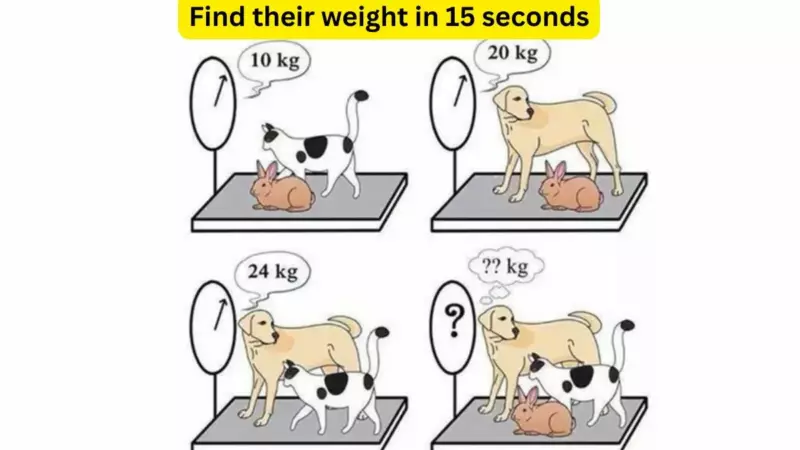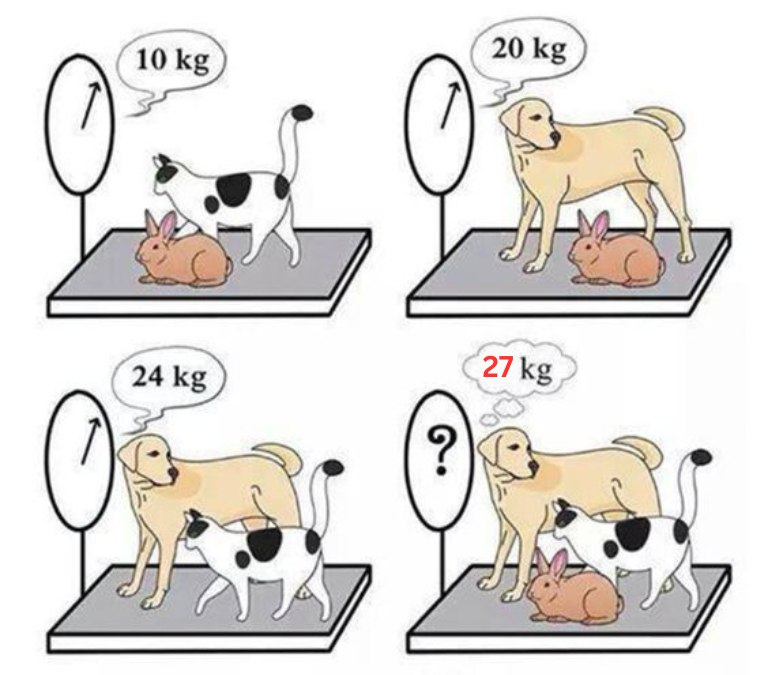# Test your IQ. Can you find the weight of cat, dog, and rabbit in 15 seconds!interesting stories

In the image above, you can see a cat, a dog, and a rabbit on weighing scales.The first scale reads the weight of the cat and the dog. Their combined weight is 10 kilogrammes.

The second scale reads the weight of the dog and the rabbit. Their combined weight is 20 kilogrammes.The third scale reads the weight of the dog and the cat. Their combined weight is 24 kilogrammes.

The fourth scale is weighing all three animals- the cat, the dog, and the rabbit- but, their combined weight is not known. This is what you have to find out.

What is the combined weight of the cat, the dog, and the rabbit?All the best!Now, C + R = 10D + R = 20

D + C = 24

C + D + R = ???

Let’s use the first equation and find the value of C.

C = 10 — R

Moving on to the second equation, let’s find the value of D.

D = 20 — R

Now, substitute the values of C and D in the third equation.

(20 — R) + (10 — R) = 24

=> 20 + 10 — R — R = 24

=> 30 — 2R = 24

=> 6 = 2R

=> 3 = R

So, the weight of the rabbit is 3 kg.

Substitute the weight of the rabbit in the last equation.

(C + D) + R = ?

=> 24 + 3 = ?

=> 27

So, the combined weight of the cat, dog, and rabbit is 27 kg.

(Visited 78 times, 3 visits today)
Оцените статью